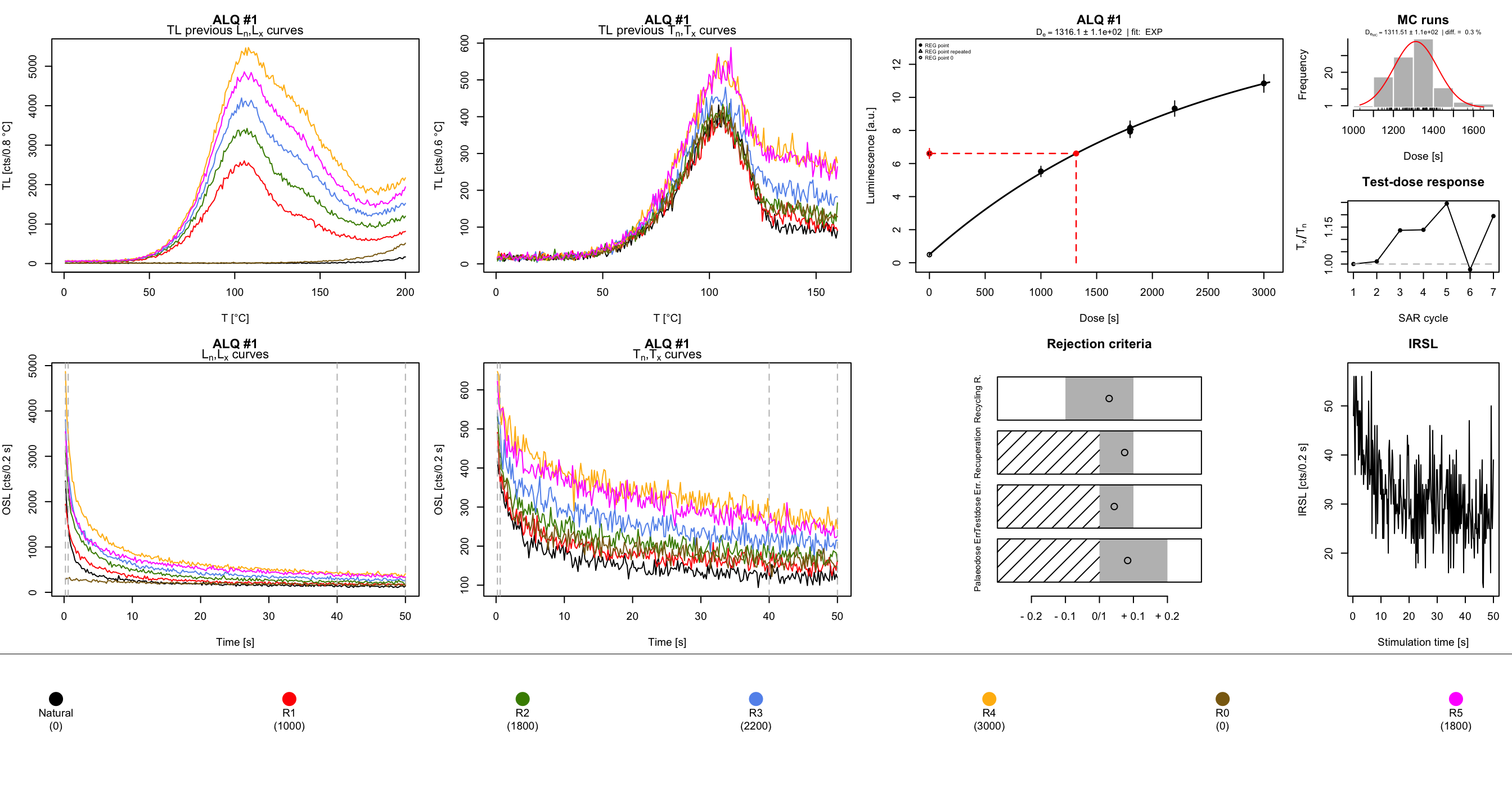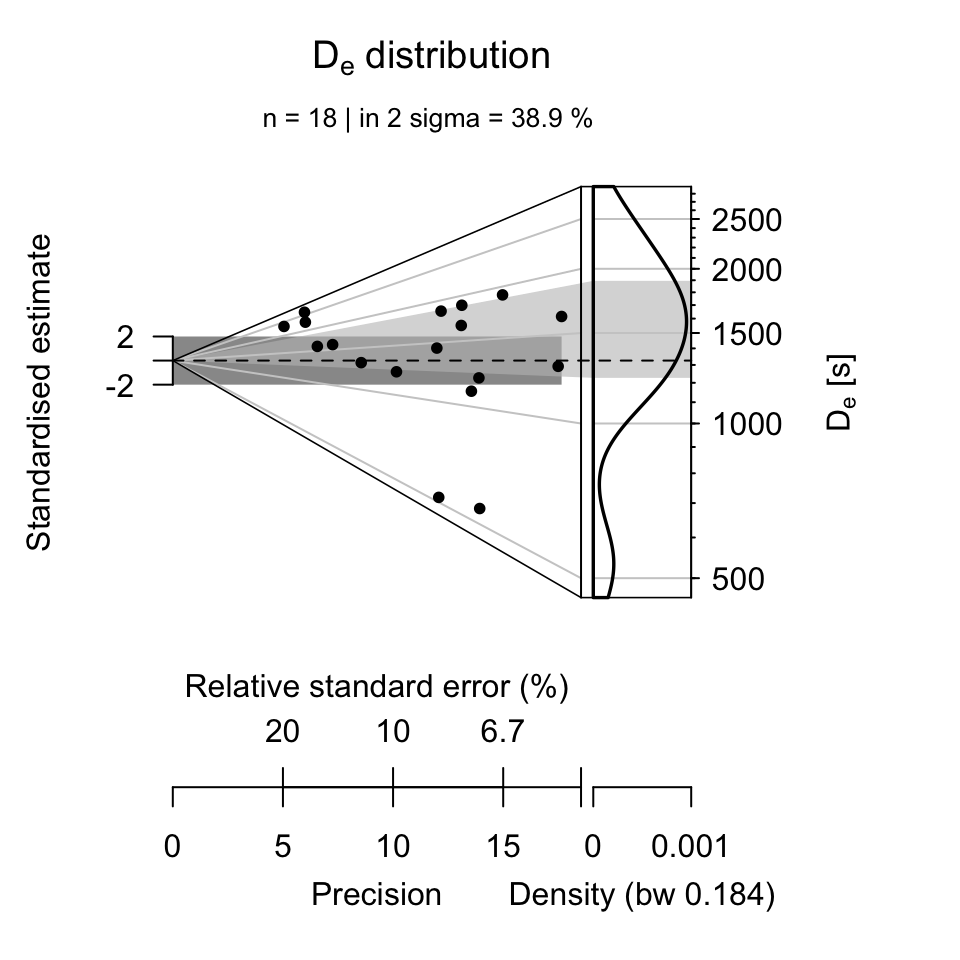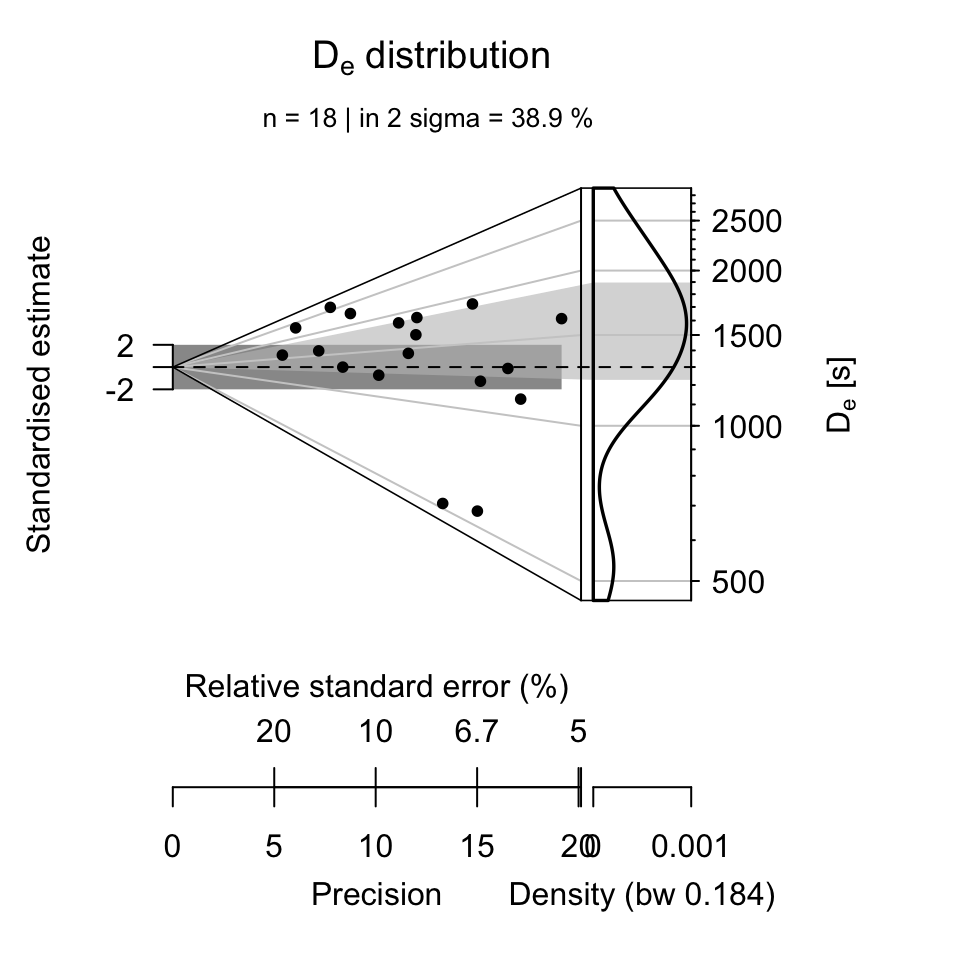## It takes only 4 steps to analyse your SAR luminescence data

###### by Sebastian Kreutzer & R Luminescence Team (February 21, 2020)
[last updated: 2021-08-02]

Luminescence >= 0.6.0Creative Commons

Usually R does not cause a lot of frustration, but only if you know how to use it. However, first steps are difficult. To ease the beginning for analysing luminescence data, in 2015 we published a guide to analyse SAR data. Since then, ’Luminescence' evolved, today making the luminescence data analysis easier than ever before. By using the example data (SAR, quartz) published along with the article by , here again we demonstrate how to analyse the luminescence data with R. In only 4 steps, promised, it was never easier.

## Step 1: Load the package ‘Luminescence’

Simple, but indispensable, our first step is loading the R package ’Luminescence':

library(Luminescence)

Without data, no data analysis. We now import the measurement data into R by calling the function read_BIN2R() (if you have XSYG-files, use read_XSYG2R() instead). The function file.choose() opens an external window to allow are very convenient file selection. The argument fastForward makes sure that the data are directly available in the format we want to have them.

file <- file.choose()
temp <- read_BIN2R(file, fastForward = TRUE)

Not every data set is instantly ready to be analysed, e.g., the data set may contain additional information, which are not needed for the analysis. This is the case for our test data set. As written in , only the first 20 aliquots follow the common SAR procedure, i.e. a consecutive sequence of irradiation, heating, OSL ($$L_x$$), irradiation, heating and OSL ($$T_x$$). Therefore, we have to limit our data set to the first 20 aliquots:

temp.sel <- temp[1:20]

Note: Further limitations can be applied by using the function get_RLum(). Type ?get_RLum in your R console to get further information. Please do not forget to set the parameter get_RLum(...,drop = FALSE) otherwise your object will look different from what the function expects as input.

## Step 3: Run the SAR analysis

Now the data are ready and can be analysed calling the function analyse_SAR.CWOSL:

results <- analyse_SAR.CWOSL(
object = temp.sel,
signal.integral.min = 1,
signal.integral.max = 5,
background.integral.min = 200,
background.integral.max = 250,
fit.method = "EXP",
rejection.criteria = list(
recycling.ratio = 10,
recuperation.rate = 10,
testdose.error = 10,
palaeodose.error = 20,
exceed.max.regpoint = TRUE),
plot_onePage = TRUE
)Figure 1: Example output for one aliquot.

The graphical output consists of three different plot sets: (A) the preheat curves (here as TL) and the OSL curves used to calculate $$L_{x}/T_{x}$$, (B) the dose response curve and (C) a plot showing visualising the rejection criteria and, if measured, the IRSL curve checking for a potential feldspar contamination of the quartz sample.

To modify arguments or to understand the meaning of the given arguments, please type ?analyse_SAR.CWOSL in your R console.

The results of the analysis is a complex R object (here named results), which can be used for further analysis.

results
##
##  [RLum.Results-class]
##   originator: analyse_SAR.CWOSL()
##   data: 4
##       .. $data : data.frame ## ..$LnLxTnTx.table : data.frame
##   .. $rejection.criteria : data.frame ## ..$Formula : list
##   additional info elements:  20

To show only the obtained equivalent dose $$D_{e}$$ values, the following line needs to be typed on the R console:

head(results$data) ## De De.Error D01 D01.ERROR D02 D02.ERROR Dc De.MC Fit HPDI68_L ## 1 1195.3066 72.40373 2651.342 842.4220 NA NA NA 1205.908 EXP 1114.832 ## 2 517.3256 45.62979 1759.007 317.8390 NA NA NA 519.418 EXP 463.140 ## 3 1586.1348 254.24673 1193.753 326.5909 NA NA NA 1641.946 EXP NA ## 4 2594.8597 430.28344 2053.184 638.2911 NA NA NA 2661.868 EXP NA ## 5 1098.9140 74.76314 2361.560 748.5487 NA NA NA 1116.073 EXP 1028.309 ## 6 1445.5594 140.54190 1888.343 688.1423 NA NA NA 1458.003 EXP 1314.198 ## HPDI68_U HPDI95_L HPDI95_U RC.Status signal.range background.range ## 1 1262.8303 1068.9343 1363.0568 OK 1 : 5 200 : 250 ## 2 563.9779 434.3291 611.2277 OK 1 : 5 200 : 250 ## 3 NA NA NA OK 1 : 5 200 : 250 ## 4 NA NA NA OK 1 : 5 200 : 250 ## 5 1181.2135 979.9076 1276.9163 OK 1 : 5 200 : 250 ## 6 1604.2356 1176.5887 1748.1959 OK 1 : 5 200 : 250 ## signal.range.Tx background.range.Tx UID ## 1 NA : NA NA : NA 2021-08-02-03:04.0.739523517899215 ## 2 NA : NA NA : NA 2021-08-02-03:04.0.708020005607978 ## 3 NA : NA NA : NA 2021-08-02-03:04.0.338453078176826 ## 4 NA : NA NA : NA 2021-08-02-03:04.0.882017500931397 ## 5 NA : NA NA : NA 2021-08-02-03:04.0.227275899378583 ## 6 NA : NA NA : NA 2021-08-02-03:04.0.679579654941335 ## Step 4: Plot your data The last step. No luminescence data analysis without a graphical representation of the results. Below we have chosen the abanico plot to visualise the final $$D_e$$ distribution. The prior used function subset ensures that only values are shown, which have successfully been passed the rejection criteria. df <- subset(results$data, RC.Status == "OK")
plot_AbanicoPlot(df[,1:2], zlab = expression(paste(D[e], " [s]")))# The compact call

Now let’s do all of this in a single, neat call using the |> pipe operator supported in R >= 4.0.0 onwards.

Note: Minor differences in the plot are due to the Monte Carlo runs used for the estimation of the uncertainty.

results <- read_BIN2R(file, verbose = FALSE, fastForward = TRUE)[1:20] |>
analyse_SAR.CWOSL(
signal.integral.min = 1,
signal.integral.max = 5,
background.integral.min = 200,
background.integral.max = 250,
fit.method = "EXP",
rejection.criteria = list(
recycling.ratio = 10,
recuperation.rate = 10,
testdose.error = 10,
palaeodose.error = 20,
exceed.max.regpoint = TRUE),
plot = FALSE,
verbose = FALSE) |>
get_RLum(data.object = "data") |>
subset(RC.Status == "OK") |>
plot_AbanicoPlot(zlab = expression(paste(D[e], " [s]")))